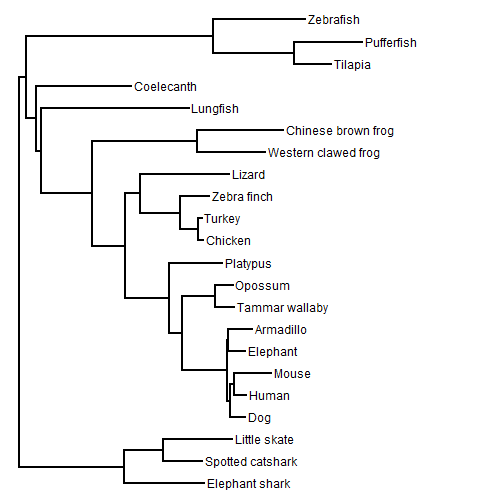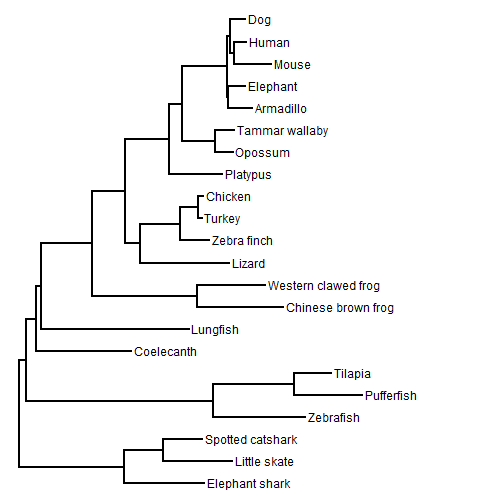## Tuesday, January 31, 2017

### New tree drawing method to extract phylogeny from a plotted figure

After yesterday posting a video illustrating how to build a tree by hand using `bind.tip` in the phytools package, it occurred to me that the next logical step would be a function that would allow the user to build a tree completely free-hand, including with edge lengths, on top of an image of a tree.

The following is some code that will do this, below which I've posted another video demo. For the example I have used the tree from Amemiya et al. (2013).

``````library(phytools)
library(jpeg)
get.treepos<-phytools:::get.treepos

tree.drawer<-function(img){
par(fg=make.transparent("grey",0.8))
plot.new()
par(mar=rep(0.1,4))
plot.window(xlim=c(0,10),ylim=c(0,10))
rasterImage(img,0,0,10,10)
cat("  Click the position of the GLOBAL ROOT.\n")
flush.console()
root<-unlist(locator(1))
cat("  Enter the name of a tip RIGHT of the root. > ")
flush.console()
cat(paste("  Click on the position of ",right,".\n",sep=""))
flush.console()
right.xy<-unlist(locator(1))
cat("  Enter the name of a tip LEFT of the root. > ")
flush.console()
cat(paste("  Click on the position of ",left,".\n",sep=""))
flush.console()
left.xy<-unlist(locator(n=1))
left.xy
tree<-list(edge=matrix(c(3,3,1,2),2,2),
edge.length=c(right.xy-root,left.xy-root),
Nnode=1,tip.label=c(right,left))
class(tree)<-"phylo"
tips<-setNames(c(right.xy,left.xy),tree\$tip.label)
names(tips)<-gsub(" ","_",names(tips))
color=make.transparent("blue",0.4),lwd=4)
tip<-0
cat("  Enter the name of tip to add (or press ENTER). > ")
flush.console()
while(tip!=""){
cat(paste("  Click on the position of ",tip,".\n",sep=""))
flush.console()
xy<-unlist(locator(1))
cat("  Click on the position of its MRCA in the built tree.\n")
flush.console()
obj<-get.treepos(message=FALSE)
tree<-bind.tip(tree,tip,edge.length=xy-(nodeheight(tree,obj\$where)-
obj\$pos),where=obj\$where,position=obj\$pos)
tips<-c(tips,setNames(xy,tip))
names(tips)<-gsub(" ","_",names(tips))
plot.new()
par(mar=rep(0.1,4))
plot.window(xlim=c(0,10),ylim=c(0,10))
rasterImage(img,0,0,10,10)
color=make.transparent("blue",0.4),lwd=4)
old<-tip
cat("  Enter the name of tip to add (or press ENTER). > ")
flush.console()
while(tip=="GOBACK"){
cat(paste("  Dropping ",old,".\n",sep=""))
tree<-drop.tip(tree,old)
plot.new()
par(mar=rep(0.1,4))
plot.window(xlim=c(0,10),ylim=c(0,10))
rasterImage(img,0,0,10,10)
color=make.transparent("blue",0.4),lwd=4)
cat("  Enter the name of tip to add (or press ENTER). > ")
flush.console()
}
}
par(fg="black")
tree
}

tree<-tree.drawer(img="Amemiya_etal_2013-tree.jpg")
``````

Here is our final tree:

``````plotTree(tree)
``````The result, of course, is an object of class `"phylo"` that we can write to file or use in any other analysis that we might be interested in.

So far this only works for right-facing phylograms, but in principle one could extend to any plotting style - with a little work.

Astute readers may notice that the node rotations of the final tree are different than in the original tree - although the topology & branch lengths are correct. Well, should we be so inclined, we can 'fix' this using `tipRotate` as follows:

``````tip.order<-c("Elephant_shark","Little_skate","Spotted_catshark","Zebrafish",
"Pufferfish","Tilapia","Coelecanth","Lungfish","Chinese_brown_frog",
"Western_clawed_frog","Lizard","Zebra_finch","Turkey","Chicken","Platypus",
tree<-tipRotate(tree,setNames(1:Ntip(tree),tip.order))
plotTree(tree)
``````Neat.

1.Hi,
I would like to know more information about the function, if was incorporated in a new version of the phytools package under another name? if have been used/cited before? or if you have any software/package suggestion for extracting trees?
Ernesto

1.Ernesto. This function is now in the package physketch which can be installed from GitHub using devtools as follows:

library(devtools) ## with devtools installed
install_github("liamrevell/physketch")

- Liam

2.Hi Liam,

Given that you do not tell the function about the scale bar, is it preserving the original branch lengths?

Note: due to the very large amount of spam, all comments are now automatically submitted for moderation.